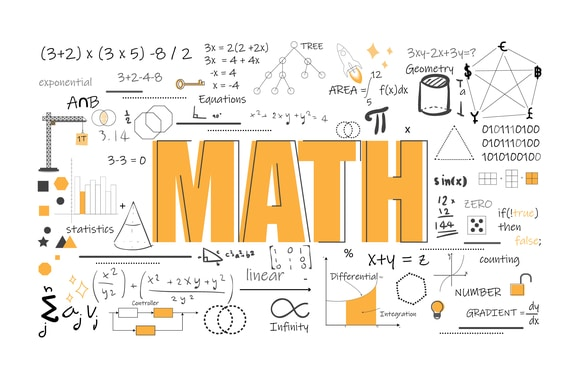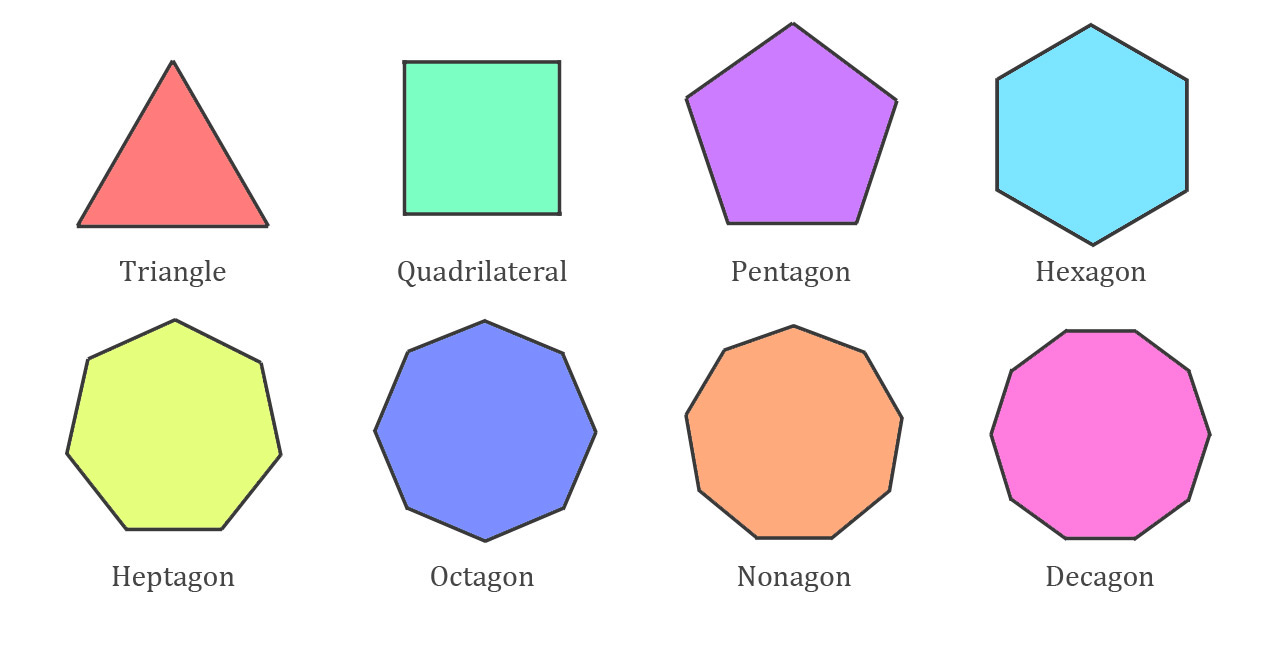# Angles and Polygons

Welcome to JSS2 Third Term!

In today’s Mathematics class, We will be discussing Angles and Polygons. We hope you enjoy the class!

CONTENT:    (i) Sum of interior angles of a polygon

(ii) Sum of exterior angles of a polygon##### DEFINITION OF A POLYGON

A polygon is any close plane figure with a straight side. A regular polygon has all sides and angles equal.

Polygons are named according to the number of sides they have. Examples are:

Triangle                     a 3- sided polygon

Pentagon                 a 5- sided polygon

Hexagon                  a 6- sided polygon

Heptagon                a 7- sided polygon

Octagon                  an 8- sided polygon

Nonagon                 a 9- sided polygon

Decagon                 a 10- sided polygon

The diagrams below represent some common polygons.Reference

NGM Book 2

Essential Mathematics for junior secondary school Book 2, chapter 9, pages 87 – 88

##### Sum of Interior Angles of a Polygon

The angles inside a polygon are called its interior angles as shown in the figure below:The number of triangles depends on the number of sides of the polygon. For a polygon with ‘n’ sides, there will be (n-2) triangles. The sum of angles of a triangle is 1800.

Alternatively, since 1800 = (n-2) x 2 x 90

= 2(n-2) 90

= (2n-4) 90

Thus, the sum of the angles of an n-sided polygon can be represented as (n-2) 1800 or (2n-4) 900

The table below shows the sum of interior angles of a regular polygon of a 3 sided polygon up to a sided polygon.

 Polygon No. of Sides No. of Triangles (n-2) Sum of interior Angles (n-2) 1800 Triangle 3 3 – 2 = 1 1 x 1800 = 180 Quadrilateral 4 4 – 2 = 2 2 x 1800 = 3600 Pentagon 5 5 – 2 = 3 3 x 1800 = 5400 Hexagon 6 6 – 2 = 4 4 x 1800 = 7200 Heptagon 7 7 – 2 = 5 5 x 1800 = 9000 Octagon 8 8 – 2 = 6 6 x 1800 = 10800 Nonagon 9 9 – 2 = 7 7 x 1800 = 12600 Decagon 10 10 – 2 = 8 8 x 1800 = 14400

Worked Examples:

1. Calculate the size of an exterior angle of a regular nonagon (9 sides)
2. Calculate the size of an exterior angle of a regular hexagon (6 sides)

1. Sum of the exterior of a polygon = 3600. Number of sides of a nonagon = 9

1. Sum of exterior angles = 360o

Hexagon has 6 sides.

= 60o

GENERAL EVALUATION

1. The interior angles of a triangle add up to …………………………
3. The sum of the interior angles of a regular polygon is 1080o. How many sides has the polygon?

REVISION QUESTION

1. Calculate the number of sides of each of a regular polygon whose interior angle is 162o
2. The sum of the 3 angles of a hexagon is 345o. If the other angles are equal. Find the sizes of each of the angle.

Essential Mathematics for junior secondary school Book 2, Chapter 19, page 252 – 255

Exercise 19.5 No 1 page 255

WEEKEND ASSIGNMENT

1. The sum of the interior angles of a regular pentagon is  A. 240o      B. 720o    C. 540o     D. 640o
2. Calculate the size of each exterior angle of a regular hexagon. A. 60o    B. 30o     C. 45o     D. 125o
3. The size of each angle of a regular octagon will be ____ A. 95o  B. 75o     C. 105o     D. 135o
4. How many sides has a polygon if the sum of interior angles of that polygon gives 3240o? A. 18o    B. 15o      C. 17o     D. 20o
5. Calculate the size of each exterior angles of a pentagon A. 30o    B. 72o      C. 60o     D. 90o

THEORY

1. Calculate
• A. The total internal angels of an octagon
• B. The size of each angle of a regular octagon
2. Calculate the
• Exterior angle
• The number of sides of a regular polygon with an interior angle of 72o

We have come to the end of this class. We do hope you enjoyed the class?

Should you have any further question, feel free to ask in the comment section below and trust us to respond as soon as possible.

In our next class, we will be talking about “Angles of Elevation and Depression”. We are very much eager to meet you there.

Are you a Parent? Share your quick opinion and win free 2-month Premium Subscription

### 5 thoughts on “Angles and Polygons”

1.I don’t understand the number 2 theory question
thanks

2.PLEASE PERMIT ME TO COPY .

3.pls can you give me and explain the answer in the NGM page 45 chapter 9 revision excersise 6 my daughter needs it pls

4.pls can you explain chapter 6 page 45 revision excersise 6 my daughter needs it

Don`t copy text!